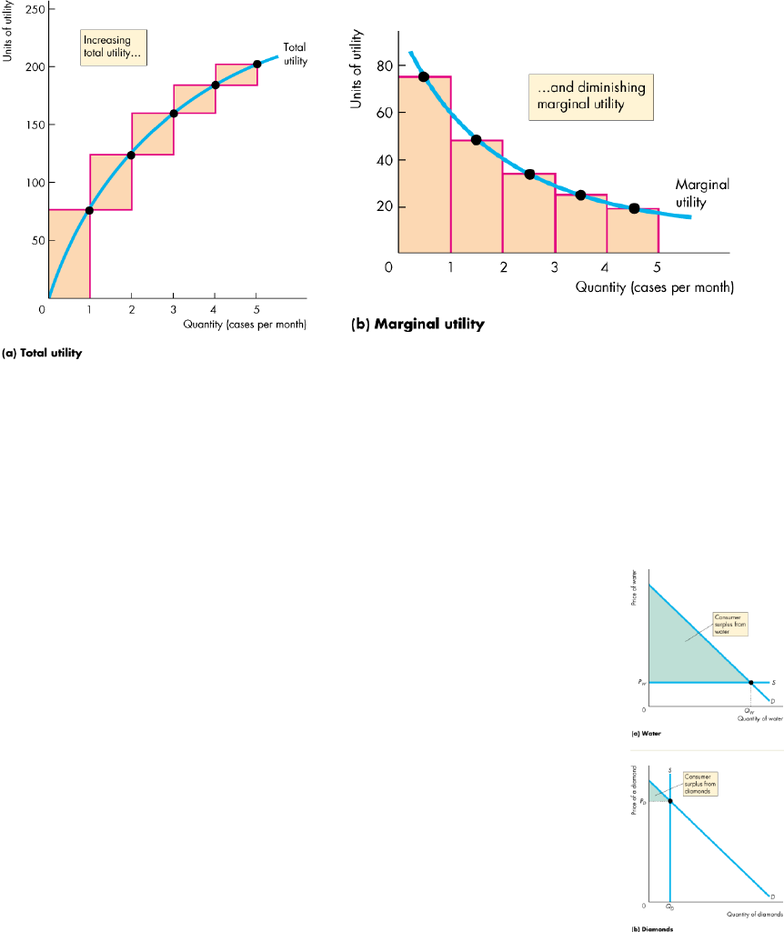# ECON 1000 Lecture Notes - Marginal Utility, Economic Surplus, Indifference Curve

47 views5 pages
Page:
of 5Ch. 8: Utility and Demand
Maximizing Utility
utility: the benefit or satisfaction that a person gets from the consumption of goods and
services
o total utility: the total benefit that a person gets from the consumption of all the
different goods and services; generally more consumption gives more total utility
o marginal utility: the change in total utility that results from a one-unit increase in the
quantity of a good consumed
diminishing marginal utility: decrease in marginal utility as the quantity of a
good consumed increases
Consumer equilibrium: utility-maximizing combination of two or more products
Choosing at the margin: consumer’s total utility is maximized by the following rule
o spend all the available income
o equalize the marginal utility per dollar for all goods
marginal utility per dollar: marginal utility divided by price
marginal utility from a good obtained by spending one more dollar
utility maximizing rule
o MU1 and MU2 are the marginal utilities of two products
o P1 and P2 are the prices of the tow products
o MU1/P1 = MU2/P2
o MU1 = MU2 x P1/P2
o Why is the price of an essential cheaper than the price of a luxury?
Water has low marginal utility but high total utility
Diamonds have high marginal utility but low total utility
Marginal utility per dollar is the same
o Water supply is perfectly elastic so quantity consumed is large and consumer surplus is
large
o Diamond supply is perfectly inelastic so price is high and consumer surplus is low
Ch. 9: Possibilities, Preferences, and Choices
Consumption Possibilities
Budget line: describes the limits to a household’s consumption choices
o any point left of or on the budget line is affordable
o any point to the right of the budget line is unaffordable
o like a PPF but for consumption
changes in price and changes in income shifting the budget line
Budget equation
o Expenditure = Income
o P1Q1 + P2Q2 = Y
Q1 = Y/P1 Q2(P2/P1)
o Real income: income expressed as a quantity of goods that a household can afford to
At the point where the budget line intersects the y-axis
=Y/P1
o Relative price: the price of one good divided by the price of another good
=P2/P1
Opportunity cost
Preferences and Indifference Curves
Indifference curve: a line that shows combinations of goods among which a consumer is
indifferent
o any point on indifference curve is preferred to any point below an indifference curve
o any point above the indifference curve is preferred to any point on the indifference
curve
preference map: a series of indifference curves
marginal rate of substitution (MRS): the rate at which a person will give up good y to get an
additional unit of good x while remaining indifferent (on the same curve)
o tangent: magnitude of the slope of an indifference curve measures the MRS
steep slope = willingness to give up a lot of y to get an extra unit of x
flatter slope = willingness to give up little y to get an extra unit of x
o diminishing marginal rate of substitution: general tendency for a person to be willing to
give up less of good y to get one more unit of good x, while at the same time remaining
indifferent as the quantity of x increases
degree of substitutability
o shape of the indifference curve reveals the degree of substitutability
Predicting Consumer Choices
Best affordable choice
o On the budget line
o On the highest attainable indifference curve
o Marginal rate of substitution equals relative price
Price effect: the effect of the change in the price on the quantity of a good consumed
o Movement between best affordable choices on different indifference curves due to
price effect mirrors the demand curve
Income effect: the effect of the change in income on the quantity of a good consumed
o Movement between best affordable choice on different indifference curves (parallel
budget lines) is a shift of a demand curve